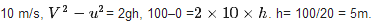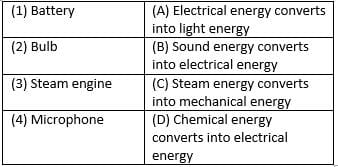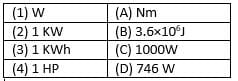HOTS Questions: Work & Energy

# HOTS Questions: Work & Energy - Class 9

Test Description

## 25 Questions MCQ Test Science Class 9 - HOTS Questions: Work & Energy

HOTS Questions: Work & Energy for Class 9 2023 is part of Science Class 9 preparation. The HOTS Questions: Work & Energy questions and answers have been prepared according to the Class 9 exam syllabus.The HOTS Questions: Work & Energy MCQs are made for Class 9 2023 Exam. Find important definitions, questions, notes, meanings, examples, exercises, MCQs and online tests for HOTS Questions: Work & Energy below.
Solutions of HOTS Questions: Work & Energy questions in English are available as part of our Science Class 9 for Class 9 & HOTS Questions: Work & Energy solutions in Hindi for Science Class 9 course. Download more important topics, notes, lectures and mock test series for Class 9 Exam by signing up for free. Attempt HOTS Questions: Work & Energy | 25 questions in 25 minutes | Mock test for Class 9 preparation | Free important questions MCQ to study Science Class 9 for Class 9 Exam | Download free PDF with solutions
 1 Crore+ students have signed up on EduRev. Have you?
HOTS Questions: Work & Energy - Question 1

### Work done by centripetal force is

Detailed Solution for HOTS Questions: Work & Energy - Question 1

When a body moves on a circular path. The force that acts on the revolving body towards the center is called centripetal force. As the force acts as right angle to the direction of motion, so work done is 0.

HOTS Questions: Work & Energy - Question 2

### Statement A: Any physical or mental activity is termed as work done in physics. Statement B: Work is said to be + ve work done when the body is displaced in the direction of applied force. Which of the two statements is true?

Detailed Solution for HOTS Questions: Work & Energy - Question 2

In physics, a work is said to be done when there is some displacement on the body on which force is applied. Work done is +ve when the body is displaced in direction of applied force.

HOTS Questions: Work & Energy - Question 3

### P. E of your body is maximum when you

Detailed Solution for HOTS Questions: Work & Energy - Question 3

Potential Energy of a body = mgh, hence the potential energy is maximum when you are standing, given that acceleration due to gravity and mass remain constant.

HOTS Questions: Work & Energy - Question 4

According to the law of conservation of energy

Detailed Solution for HOTS Questions: Work & Energy - Question 4

According to the law of conservation of energy, energy neither be created nor be destroyed, it can be transformed from one form to another. So, all the statements are true.

HOTS Questions: Work & Energy - Question 5

For what value of .ϕ. is work done maximum?

Detailed Solution for HOTS Questions: Work & Energy - Question 5

Work done when a body moves at an angle to the direction of force is W=Fcosϕ×s. The value of Cos is maximum for 00. So, work done will be maximum for this angel.

HOTS Questions: Work & Energy - Question 6

Find the correct statement

Detailed Solution for HOTS Questions: Work & Energy - Question 6

One Giga watt is equal to 109 watt or 106 kilowatt. The rate of doing work is called as power. Hence both statements are correct.

HOTS Questions: Work & Energy - Question 7

Statement A: An aeroplane flying at an altitude possesses only K. E.
Statement B: An aeroplane flying at an altitude possesses both K. E and P. E.
Which of the two statement is true

Detailed Solution for HOTS Questions: Work & Energy - Question 7

An aeroplane flying at an altitude possesses both kinetic energy and potential energy. Kinetic energy is due to mass and velocity and potential energy is due to mass and height.

HOTS Questions: Work & Energy - Question 8

A ball is dropped from a height ‘h’. The velocity of the ball when it reaches the ground is 10 m/s. Find ‘h’ (g = 10ms−2)

Detailed Solution for HOTS Questions: Work & Energy - Question 8

Initial velocity (u)= 0, final velocity (v) =HOTS Questions: Work & Energy - Question 9

1k w h = ?

Detailed Solution for HOTS Questions: Work & Energy - Question 9

1 kwH = 1000 W×60×60 s = 3600000J = 3.6 × 106  J. one watt is equal to one joule per second.

HOTS Questions: Work & Energy - Question 10

An electric lamp of 100w is used for 5 hours per day calculate the units of energy consumed by lamp in one day

Detailed Solution for HOTS Questions: Work & Energy - Question 10

Power of lamp = 100w = 100/1000 = 0.1Kw, time= 5 hrs. Electrical energy = power×timepower×time, E = 0.1kw×5h = 0.5 kw–h. = 0.5 unit.

HOTS Questions: Work & Energy - Question 11

Find the incorrect statement

Detailed Solution for HOTS Questions: Work & Energy - Question 11

The potential energy of a freely falling object decreases progressively due to decrease in height. Kinetic energy is converted into heat and sound energy when a freely falling object on reaching the ground. Work is done only when change in position occurs.

HOTS Questions: Work & Energy - Question 12

Statement A: Mechanical energy of a system is always conserved.
Statement B: The total energy of the system is always conserved.
Which of the two statements is true?

Detailed Solution for HOTS Questions: Work & Energy - Question 12

The total energy of the system is always conserved. It is just transformed from one form to other form. Mechanical energy of a system is not conserved. It is converted into other form of energy.

HOTS Questions: Work & Energy - Question 13

1 W. S = ________?

Detailed Solution for HOTS Questions: Work & Energy - Question 13

1 watt second (W.S) = 1J/s×s= 1 joule.

HOTS Questions: Work & Energy - Question 14

In which of the following activities the work is said to be done?

Detailed Solution for HOTS Questions: Work & Energy - Question 14

No work is done in reading a book, pulling wall of house and walking on a level road with book on his head as there is no change in the position of object on which force is applied. Stretching string causes displacement, so it is a work.

HOTS Questions: Work & Energy - Question 15

When you compress a coil spring you do work on it. The elastic P. E energy

Detailed Solution for HOTS Questions: Work & Energy - Question 15

When you compress a coil spring you do work on it. The elastic potential energy increases.

HOTS Questions: Work & Energy - Question 16

Match the following with correct response.HOTS Questions: Work & Energy - Question 17

A certain household has consumed 250 units of energy during a month. How much energy is this in joules?

Detailed Solution for HOTS Questions: Work & Energy - Question 17

1 unit (Kw-H) = 3.6×106 joule. Therefore, 250 units = 250×3.6×106joule
= 9×108 Joule.

HOTS Questions: Work & Energy - Question 18

Which of the following is a non metal that remains liquid at room temperature?

Detailed Solution for HOTS Questions: Work & Energy - Question 18

It is bromine as it has boiling point of 58.78 and has -7.2 melting point.

HOTS Questions: Work & Energy - Question 19

P.E of a spring increases when it is in the state of

Detailed Solution for HOTS Questions: Work & Energy - Question 19

Potential energy of a spring increases when it is in the state of expansion and compression. Elastic potential energy increases with compression and gravitational potential energy increases on expansion.

HOTS Questions: Work & Energy - Question 20

Work done by the gravitational force of earth on a satellite along a circular path is

Detailed Solution for HOTS Questions: Work & Energy - Question 20

The gravitational force of the earth on a satellite along a circular path is O as gravitational force of earth acts on right angle which is O for cos.

HOTS Questions: Work & Energy - Question 21

9800 joule of energy was spent to raise a mass of 50kg, the mass was raised to a height of

Detailed Solution for HOTS Questions: Work & Energy - Question 21

Potential energy = mgh, here, RE = 9800 j. mass = 50kg, g = 9.8m/s2. Therefore, 9800 = 50 x 9.8 x h, h= 9800/50 x 9.8 = 20m.

HOTS Questions: Work & Energy - Question 22

Match the following with correct response.HOTS Questions: Work & Energy - Question 23

What happens to the kinetic energy of a freely falling object which eventually stops on reaching the ground?

Detailed Solution for HOTS Questions: Work & Energy - Question 23

The kinetic energy of a freely falling object which eventually stops on reaching the ground changes into heat and sound energy.

HOTS Questions: Work & Energy - Question 24

The linear momentum of a body of mass 2kg is 16kgms−1. What is its K. E?

Detailed Solution for HOTS Questions: Work & Energy - Question 24

Momentum = mass x velocity, velocity = momentum/ mass = 16/2 = 8m/s. Kinetic energy = ½ mv2. K.E = ½ x2 x 82 = 64 J.

HOTS Questions: Work & Energy - Question 25

Which of the following is unit of energy
A. Newton metre
B. Joule
C. Kilowatt hours
D. Kilowatt

Detailed Solution for HOTS Questions: Work & Energy - Question 25

We know that, joule, newton metre and kilowatt hour are the units of energy and the kilowatt is the unit of power.

## Science Class 9

66 videos|352 docs|97 tests
Information about HOTS Questions: Work & Energy Page
In this test you can find the Exam questions for HOTS Questions: Work & Energy solved & explained in the simplest way possible. Besides giving Questions and answers for HOTS Questions: Work & Energy , EduRev gives you an ample number of Online tests for practice

## Science Class 9

66 videos|352 docs|97 tests